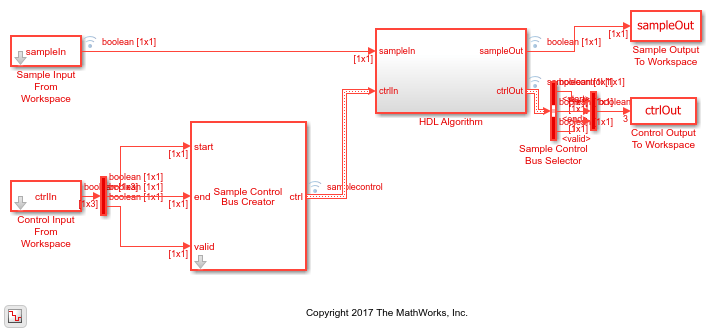# Append CRC Checksum to Streaming Data

This example shows how to use the LTE CRC Encoder block to encode data, and how to compare the hardware-friendly design with the results from LTE Toolbox™. The workflow follows these steps:

1. Generate frames of random input samples in MATLAB.

2. Generate and append a CRC checksum using the LTE Toolbox function `lteCRCEncode`.

3. Convert framed input data to a stream of samples and import the stream into Simulink®.

4. To encode the samples using a hardware-friendly architecture, run the Simulink model, which contains the Wireless HDL Toolbox™ block LTE CRC Encoder.

5. Export the stream of bits, which now has an appended CRC checksum, to the MATLAB® workspace.

6. Convert the sample stream back to framed data, and compare the frames with the reference frames and checksum.

Generate input data frames. Generate reference output data using `lteCRCEncode`.

```frameLength = 256; numframes = 2; rng(0); txframes = cell(1,numframes); txcodeword = cell(1,numframes); rxSoftframes = cell(1,numframes); for ii = 1:numframes txframes{ii} = randi([0 1],frameLength,1)>0.5; CRCType = '24B'; CRCMask = 50; txcodeword{ii} = lteCRCEncode(txframes{ii},CRCType,CRCMask); end ```

Serialize input data for the Simulink model. Leave enough time between frames for each frame to be fully encoded before the next one starts. For CRC 24 encoding, the checksum adds 24 parity bits at the end of the frame. The hardware-friendly algorithm also adds CRCLength + 3 cycles of latency.

```idleCyclesBetweenSamples = 0; idleCyclesBetweenFrames = 24+27; outputSize = 1; [sampleIn,ctrlIn] = whdlFramesToSamples(... txframes,idleCyclesBetweenSamples,idleCyclesBetweenFrames,outputSize); ```

```sampletime = 1; simTime = length(ctrlIn); modelname = 'ltehdlCRCEncoderModel'; open(modelname); sim(modelname); ```The Simulink model exports `sampleOut` and `ctrlOut` back to the MATLAB workspace. Deserialize the output samples, and compare the framed data to the reference data.

```txhdlframes = whdlSamplesToFrames(sampleOut,ctrlOut); fprintf('\nLTE CRC Encoder\n'); for ii = 1:numframes numBitsDiff = sum(double(txcodeword{ii})-double(txhdlframes{ii})); fprintf([' Frame %d: Behavioral and ' ... 'HDL simulation differ by %d bits\n'], ii, numBitsDiff); end ```
```Maximum frame size computed to be 280 samples. LTE CRC Encoder Frame 1: Behavioral and HDL simulation differ by 0 bits Frame 2: Behavioral and HDL simulation differ by 0 bits ```